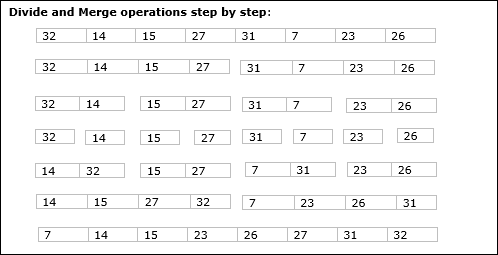• Design and Analysis of Algorithms
• Home

Design and Analysis Merge Sort

In this chapter, we will discuss merge sort and analyze its complexity.

Problem Statement

The problem of sorting a list of numbers lends itself immediately to a divide-and-conquer strategy: split the list into two halves, recursively sort each half, and then merge the two sorted sub-lists.

Solution

In this algorithm, the numbers are stored in an array numbers[]. Here, p and q represents the start and end index of a sub-array.

Algorithm: Merge-Sort (numbers[], p, r)
if p < r then
q = ⌊(p + r) / 2⌋
Merge-Sort (numbers[], p, q)
Merge-Sort (numbers[], q + 1, r)
Merge (numbers[], p, q, r)

Function: Merge (numbers[], p, q, r)
n1 = q – p + 1
n2 = r – q
declare leftnums[1…n1 + 1] and rightnums[1…n2 + 1] temporary arrays
for i = 1 to n1
leftnums[i] = numbers[p + i - 1]
for j = 1 to n2
rightnums[j] = numbers[q+ j]
leftnums[n1 + 1] = ∞
rightnums[n2 + 1] = ∞
i = 1
j = 1
for k = p to r
if leftnums[i] ≤ rightnums[j]
numbers[k] = leftnums[i]
i = i + 1
else
numbers[k] = rightnums[j]
j = j + 1

Analysis

Let us consider, the running time of Merge-Sort as T(n). Hence,

$T(n)=\begin{cases}c & if\:n\leqslant 1\\2\:x\:T(\frac{n}{2})+d\:x\:n & otherwise\end{cases}$ where c and d are constants

Therefore, using this recurrence relation,

$$T(n) = 2^i T(\frac{n}{2^i}) + i.d.n$$

As, $i = log\:n,\: T(n) = 2^{log\:n} T(\frac{n}{2^{log\:n}}) + log\:n.d.n$

$=\:c.n + d.n.log\:n$

Therefore, $T(n) = O(n\:log\:n)$

Example

In the following example, we have shown Merge-Sort algorithm step by step. First, every iteration array is divided into two sub-arrays, until the sub-array contains only one element. When these sub-arrays cannot be divided further, then merge operations are performed.Useful Video Courses

Video

Compiler Design Online Training

102 Lectures 10 hours

Video

Sequential Circuit Design

30 Lectures 3 hours

Video

Design Patterns Online Training

31 Lectures 4 hours

Video

InDesign Online Training

43 Lectures 1.5 hours

Video

Canva: Become a Graphic Designer

7 Lectures 1 hours

Video

The Ultimate Canva Graphic Design Course

54 Lectures 4 hours